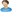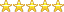Home»Mathematics and Statistics» Regression analysis of malaria in Nigeria

# Regression analysis of malaria in Nigeria

Department: Mathematics and Statistics
By:ultimeight

Project ID: 3291
Rating:(5.0) votes: 1
Rate this project
Price:₦2000
Abstract
Malaria is a life-threatening disease that is usually transmitted to people through the bite of infected female anopheles mosquitoes. However, this article deals with the regression analysis of malaria in Nigeria. Presents the incidence rate of malaria in Nigeria per 100,000 people. The study covers a period of thirteen (13) years (2007 to 2019, the incidence rate of malaria, the analysis includes correlation, fitting of regression, predicting the expected incidence rate. The negative relationship between year and incidence rate of malaria (r-0.977) and the coefficient of determination was found to be 0.954, which indicates that year was able to account for 95.4% of the variation that occurs in the incidence rate of malaria. From the fitted regression, the constant coefficient a^447.023, which indicates that the average incidence rate of malaria in Nigeria is 447 per 100,000 people, and the slope coefficient b^-15.216, which indicates that the incidence rate of malaria in Nigeria decrease every year on average, by approximately 15....
Preview +
Other Mathematics and Statistics project topics and materials you might be interested in